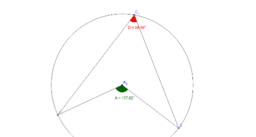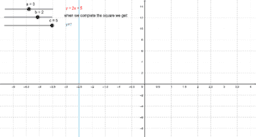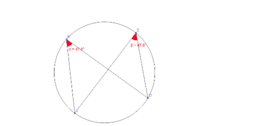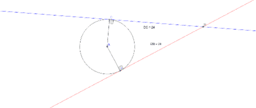# B George

•### Circle Theorem 1 - Angle at the centre = 2 x Angle at the circumference

Activity

B George

•### Circle Theorem 6 - Angles in a Cyclic Quadrilaterial

Activity

B George

•### Enlargements - Sides and Areas

Activity

B George

•### Enlargements

Activity

B George

•Activity

B George

•### Equation of a straight line - y=mx+c

Activity

B George

•### Circle Theorem 2 - Angles in the same segment are equal

Activity

B George

•### Circle Theorem 3 - Radius is Perpendicular to Tangent

Activity

B George

•### Circle Theorem 4 - Alternate angle theorem

Activity

B George

•### Circle Theorem 5 - Tangents from a point are equal length

Activity

B George

•Activity

B George